We think you are located in United States. Is this correct?

# 6.3 Reduction formula

## 6.3 Reduction formula (EMBHJ)

Any trigonometric function whose argument is $$\text{90}\text{°}±\theta$$; $$\text{180}\text{°}±\theta$$ and $$\text{360}\text{°}±\theta$$ can be written simply in terms of $$\theta$$.

## Reduction formulae for function values of $$\text{180}\text{°}±\theta$$

1. Function values of $$\text{180}\text{°}-\theta$$

In the figure $$P(\sqrt{3};1)$$ and $$P'$$ lie on the circle with radius $$\text{2}$$. $$OP$$ makes an angle $$\theta =\text{30}\text{°}$$ with the $$x$$-axis.1. If points $$P$$ and $$P'$$ are symmetrical about the $$y$$-axis, determine the coordinates of $$P'$$.

2. Write down values for $$\sin \theta$$, $$\cos \theta$$ and $$\tan \theta$$.

3. Use the coordinates for $$P'$$ to determine $$\sin(\text{180}\text{°}-θ)$$, $$\cos(\text{180}\text{°}-θ)$$, $$\tan(\text{180}\text{°}-θ)$$.

4. From your results determine a relationship between the trigonometric function values of $$\left(\text{180}\text{°}-\theta \right)$$ and $$\theta$$.

2. Function values of $$\text{180}\text{°}+\theta$$

In the figure $$P(\sqrt{3};1)$$ and $$P'$$ lie on the circle with radius $$\text{2}$$. $$OP$$ makes an angle $$\theta =\text{30}\text{°}$$ with the $$x$$-axis.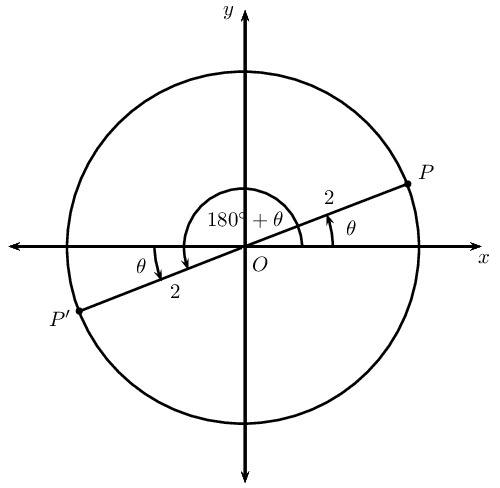1. If points $$P$$ and $$P'$$ are symmetrical about the origin (the two points are symmetrical about both the $$x$$-axis and the $$y$$-axis), determine the coordinates of $$P'$$.

2. Use the coordinates for $$P'$$ to determine $$\sin (\text{180}\text{°}+θ)$$, $$\cos (\text{180}\text{°}+θ)$$ and $$\tan (\text{180}\text{°}+θ)$$.

3. From your results determine a relationship between the trigonometric function values of $$\left(\text{180}\text{°}+\theta \right)$$ and $$\theta$$.

3. Complete the following reduction formulae:

1. $$\sin (\text{180}\text{°} - \theta) = \ldots \ldots$$
2. $$\cos (\text{180}\text{°} - \theta) = \ldots \ldots$$
3. $$\tan (\text{180}\text{°} - \theta) = \ldots \ldots$$
4. $$\sin (\text{180}\text{°} + \theta) = \ldots \ldots$$
5. $$\cos (\text{180}\text{°} + \theta) = \ldots \ldots$$
6. $$\tan (\text{180}\text{°} + \theta) = \ldots \ldots$$

## Worked example 7: Reduction formulae for function values of $$\text{180}\text{°} \pm \theta$$

Write the following as a single trigonometric ratio:

$\frac{\sin \text{163}\text{°}}{\cos \text{197}\text{°}} + \tan \text{17}\text{°} + \cos (\text{180}\text{°} - \theta) \times \tan (\text{180}\text{°} + \theta)$

### Use reduction formulae to write the trigonometric function values in terms of acute angles and $$\theta$$

$= \frac{\sin (\text{180}\text{°} - \text{17}\text{°})}{\cos (\text{180}\text{°} + \text{17}\text{°})} + \tan \text{17}\text{°} + (- \cos \theta) \times \tan \theta$

### Simplify

\begin{align*} &= \frac{\sin \text{17}\text{°}}{- \cos \text{17}\text{°}} + \tan \text{17}\text{°} - \cos \theta \times \frac{\sin \theta}{\cos \theta} \\ &= - \tan \text{17}\text{°} + \tan \text{17}\text{°} - \sin \theta \\ &= -\sin \theta \end{align*}

## Reduction formulae for function values of $$\text{180}\text{°} \pm \theta$$

Textbook Exercise 6.3

Determine the value of the following expressions without using a calculator:

$$\tan \text{150}\text{°}\sin \text{30}\text{°} - \cos \text{210}\text{°}$$

\begin{align*} &\tan \text{150}\text{°}\sin \text{30}\text{°} - \cos \text{210}\text{°} \\ &= \tan (\text{180}\text{°} - \text{30}\text{°})\sin \text{30}\text{°} - \cos (\text{180}\text{°} + \text{30}\text{°}) \\ &= - \tan \text{30}\text{°} \sin \text{30}\text{°} + \cos \text{30}\text{°} \\ &= - \left( \frac{1}{\sqrt{3}} \right) \left( \frac{1}{2} \right) + \frac{\sqrt{3}}{2} \\ &= - \frac{1}{2\sqrt{3}} + \frac{\sqrt{3}}{2} \\ &= - \frac{1}{2\sqrt{3}} \times \frac{\sqrt{3}}{\sqrt{3}} + \frac{\sqrt{3}}{2} \\ &= - \frac{\sqrt{3}}{6} + \frac{\sqrt{3}}{2} \\ &= \frac{-\sqrt{3} + 3\sqrt{3}}{6} \\ &= \frac{2\sqrt{3}}{6} \\ &= \frac{\sqrt{3}}{3} \end{align*}

$$(1 + \cos \text{120}\text{°})(1 - \sin^2 \text{240}\text{°})$$

\begin{align*} &(1 + \cos \text{120}\text{°})(1 - \sin^2 \text{240}\text{°}) \\ &= \left( 1 + \cos (\text{180}\text{°} - \text{60}\text{°}) \right) \left( 1 - \sin^2 (\text{180}\text{°} + \text{60}\text{°}) \right) \\ &= \left( 1 - \cos \text{60}\text{°} \right) \left( 1 - \sin^2 \text{60}\text{°} \right) \\ &= \left( 1 - \frac{1}{2} \right) \left( 1 - \left( \frac{\sqrt{3}}{2} \right)^2 \right) \\ &= \left( \frac{1}{2} \right) \left( 1 - \frac{3}{4} \right) \\ &= \frac{1}{2} \times \frac{1}{4} \\ &= \frac{1}{8} \end{align*}

$$\cos^2 \text{140}\text{°} + \sin^2 \text{220}\text{°}$$

\begin{align*} &\cos^2 \text{140}\text{°} + \sin^2 \text{220}\text{°} \\ &= \cos^2 (\text{180}\text{°} - \text{40}\text{°})+ \sin^2 (\text{180}\text{°} + \text{40}\text{°}) \\ &= \cos^2 \text{40}\text{°} + \sin^2 \text{40}\text{°} \\ &= \text{1} \end{align*}

Write the following in terms of a single trigonometric ratio:

$$\tan (\text{180}\text{°} - \theta) \times \sin (\text{180}\text{°} + \theta)$$

\begin{align*} &\tan (\text{180}\text{°} - \theta) \times \sin (\text{180}\text{°} + \theta) \\ &= - \tan \theta \times \left( - \sin \theta \right) \\ &= - \frac{\sin \theta}{\cos \theta} \times \left( - \sin \theta \right) \\ &= \frac{\sin^2 \theta}{\cos \theta} \\ &= \frac{1 - \cos^2 \theta}{\cos \theta} \end{align*}

$$\dfrac{\tan (\text{180}\text{°} + \theta) \cos (\text{180}\text{°} - \theta)}{\sin (\text{180}\text{°} - \theta)}$$

\begin{align*} \dfrac{\tan (\text{180}\text{°} + \theta) \cos (\text{180}\text{°} - \theta)}{\sin (\text{180}\text{°} - \theta)} &= -\dfrac{ \tan \theta \cos \theta }{\sin \theta } \\ &= -\dfrac{ \frac{\sin \theta}{\cos \theta} \cos \theta }{\sin \theta } \\ &= -\dfrac{ \sin \theta }{\sin \theta } \\ &= -\text{1} \end{align*}

If $$t = \tan \text{40}\text{°}$$, express the following in terms of $$t$$:

$$\tan \text{140}\text{°} + 3 \tan \text{220}\text{°}$$

\begin{align*} &\tan \text{140}\text{°} + 3 \tan \text{220}\text{°} \\ &= \tan (\text{180}\text{°} - \text{40}\text{°}) + 3 \tan (\text{180}\text{°} + \text{40}\text{°}) \\ &= - \tan \text{40}\text{°} + 3 \tan \text{40}\text{°} \\ &= 2 \tan \text{40}\text{°} \\ &= 2t \end{align*}

$$\frac{\cos \text{220}\text{°}}{\sin \text{140}\text{°}}$$

\begin{align*} \dfrac{\cos \text{220}\text{°}}{\sin \text{140}\text{°}} &= \dfrac{\cos (\text{180}\text{°} + \text{40}\text{°})}{\sin (\text{180}\text{°} - \text{40}\text{°})} \\ &= \dfrac{ - \cos \text{40}\text{°} }{\sin \text{40}\text{°} } \\ &= - \left( \dfrac{ \sin \text{40}\text{°} }{\cos \text{40}\text{°} } \right)^{-1} \\ &= - \left( \tan \text{40}\text{°} \right)^{-1} \\ &= - \dfrac{ 1 }{\tan \text{40}\text{°} } \\ &= - \frac{ 1 }{t} \end{align*}

## Reduction formulae for function values of ($$\text{360}\text{°} \pm \theta$$) and $$(- \theta)$$

1. Function values of ($$\text{360}\text{°} - \theta$$) and $$(- \theta)$$

In the Cartesian plane we measure angles from the positive $$x$$-axis to the terminal arm, which means that an anti-clockwise rotation gives a positive angle. We can therefore measure negative angles by rotating in a clockwise direction.

For an acute angle $$\theta$$, we know that $$-\theta$$ will lie in the fourth quadrant.

In the figure $$P(\sqrt{3};1)$$ and $$P'$$ lie on the circle with radius $$\text{2}$$. $$OP$$ makes an angle $$\theta =\text{30}\text{°}$$ with the $$x$$-axis.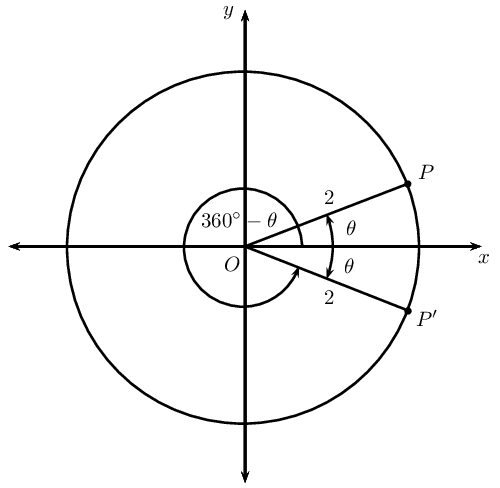1. If points $$P$$ and $$P'$$ are symmetrical about the $$x$$-axis ($$y = 0$$), determine the coordinates of $$P'$$.

2. Use the coordinates of $$P'$$ to determine $$\sin(\text{360}\text{°}-θ)$$, $$\cos(\text{360}\text{°}-θ)$$ and $$\tan(\text{360}\text{°}-θ)$$.

3. Use the coordinates of $$P'$$ to determine $$\sin (-\theta)$$, $$\cos (-\theta)$$ and $$\tan (-\theta)$$.

4. From your results determine a relationship between the function values of $$\left(\text{360}\text{°}-\theta \right)$$ and $$- \theta$$.

5. Complete the following reduction formulae:

1. $$\sin (\text{360}\text{°} - \theta) = \ldots \ldots$$
2. $$\cos (\text{360}\text{°} - \theta) = \ldots \ldots$$
3. $$\tan (\text{360}\text{°} - \theta) = \ldots \ldots$$
4. $$\sin (- \theta) = \ldots \ldots$$
5. $$\cos (- \theta) = \ldots \ldots$$
6. $$\tan (- \theta) = \ldots \ldots$$
2. Function values of $$\text{360}\text{°} + \theta$$

We can also have an angle that is larger than $$\text{360}\text{°}$$. The angle completes a revolution of $$\text{360}\text{°}$$ and then continues to give an angle of $$\theta$$.

Complete the following reduction formulae:

1. $$\sin (\text{360}\text{°} + \theta) = \ldots \ldots$$
2. $$\cos (\text{360}\text{°} + \theta) = \ldots \ldots$$
3. $$\tan (\text{360}\text{°} + \theta) = \ldots \ldots$$

From working with functions, we know that the graph of $$y = \sin \theta$$ has a period of $$\text{360}\text{°}$$. Therefore, one complete wave of a sine graph is the same as one complete revolution for $$\sin \theta$$ in the Cartesian plane.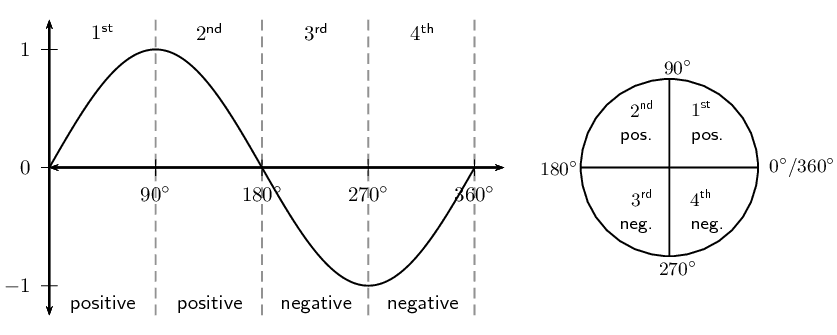We can also have multiple revolutions. The periodicity of the trigonometric graphs shows this clearly. A complete sine or cosine curve is completed in $$\text{360}\text{°}$$.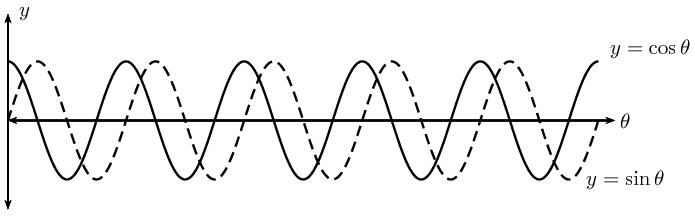If $$k$$ is any integer, then

\begin{align*} \sin (k \cdot \text{360}\text{°} + \theta) &= \sin \theta \\ \cos (k \cdot \text{360}\text{°} + \theta) &= \cos \theta \\ \tan (k \cdot \text{360}\text{°} + \theta) &= \tan \theta \end{align*}

## Worked example 8: Reduction formulae for function values of $$\text{360}\text{°} \pm \theta$$

If $$f = \tan \text{67}\text{°}$$, express the following in terms of $$f$$

$\frac{\sin \text{293}\text{°}}{\cos \text{427}\text{°}} + \tan(-\text{67}\text{°}) + \tan \text{1 147}\text{°}$

### Using reduction formula

\begin{align*} \phantom{=} \frac{\sin \text{293}\text{°}}{\cos \text{427}\text{°}} + \tan(-\text{67}\text{°}) + \tan \text{1 147}\text{°} &= \frac{\sin (\text{360}\text{°} - \text{67}\text{°})}{\cos (\text{360}\text{°} + \text{67}\text{°})} - \tan(\text{67}\text{°}) + \tan \left( 3(\text{360}\text{°}) + \text{67}\text{°} \right) \\ &= \frac{- \sin \text{67}\text{°}}{\cos \text{67}\text{°}} - \tan \text{67}\text{°} + \tan \text{67}\text{°} \\ &= - \tan \text{67}\text{°} \\ &= - f \end{align*}

## Worked example 9: Using reduction formula

Evaluate without using a calculator:

$\tan^2 \text{210}\text{°} - (1 + \cos \text{120}\text{°}) \sin^2 \text{405}\text{°}$

### Simplify the expression using reduction formulae and special angles

\begin{align*} \tan^2 \text{210}\text{°} - (1 + \cos \text{120}\text{°}) \sin^2 \text{405}\text{°} &= \tan^2 (\text{180}\text{°} + \text{30}\text{°}) - \left( 1 + \cos (\text{180}\text{°} - \text{60}\text{°}) \right) \sin^2 (\text{360}\text{°} + \text{45}\text{°}) \\ &= \tan^2 \text{30}\text{°} - \left(1 + ( - \cos\text{60}\text{°}) \right) \sin^2 \text{45}\text{°} \\ &= \left( \frac{1}{\sqrt{3}} \right)^2 - \left(1 - \frac{1}{2} \right) \left( \frac{1}{\sqrt{2}} \right)^2 \\ &= \frac{1}{3} - \left(\frac{1}{2} \right) \left( \frac{1}{2} \right) \\ &= \frac{1}{3} - \frac{1}{4} \\ &= \frac{1}{12} \end{align*}

## Using reduction formula

Textbook Exercise 6.4

Simplify the following:

$$\dfrac{ \tan (\text{180}\text{°} - \theta) \sin (\text{360}\text{°} + \theta)}{\cos (\text{180}\text{°} + \theta) \tan (\text{360}\text{°} - \theta)}$$
\begin{align*} &\dfrac{ \tan (\text{180}\text{°} - \theta) \sin (\text{360}\text{°} + \theta)}{\cos (\text{180}\text{°} + \theta) \tan (\text{360}\text{°} - \theta)} \\ &= \dfrac{ -\tan \theta \sin \theta }{(-\cos \theta )(- \tan \theta )} \\ &=-\dfrac{ \sin \theta }{ \cos \theta } \\ &= - \tan \theta \end{align*}
$$\cos^2 (\text{360}\text{°} + \theta) + \cos (\text{180}\text{°} + \theta) \tan (\text{360}\text{°} - \theta) \sin (\text{360}\text{°} + \theta)$$
\begin{align*} &\cos^2 (\text{360}\text{°} + \theta) + \cos (\text{180}\text{°} + \theta) \tan (\text{360}\text{°} - \theta) \sin (\text{360}\text{°} + \theta) \\ &= \cos^2 \theta + (- \cos \theta )(- \tan \theta) (\sin \theta ) \\ &= \cos^2 \theta + ( \cos \theta )( \frac{\sin \theta }{\cos \theta }) (\sin \theta ) \\ &= \cos^2 \theta + \sin^2 \theta \\ &= \text{1} \end{align*}
$$\dfrac{\sin (\text{360}\text{°} + \alpha) \tan (\text{180}\text{°} + \alpha)}{\cos (\text{360}\text{°} - \alpha) \tan^2 (\text{360}\text{°} + \alpha)}$$
\begin{align*} &\dfrac{\sin (\text{360}\text{°} + \alpha) \tan (\text{180}\text{°} + \alpha)}{\cos (\text{360}\text{°} - \alpha) \tan^2 (\text{360}\text{°} + \alpha)} \\ &= \dfrac{\sin \alpha \tan \alpha }{\cos \alpha \tan^2 \alpha }\\ &= \dfrac{\sin \alpha \frac{\sin \alpha}{\cos \alpha} }{\cos \alpha \frac{\sin^2 \alpha}{\cos^2 \alpha} }\\ &= \dfrac{\frac{\sin^2 \alpha}{\cos \alpha} }{ \frac{\sin^2 \alpha}{\cos \alpha} }\\ &= \dfrac{\sin^2 \alpha}{\cos \alpha} \times \frac{\cos \alpha}{\sin^2 \alpha} \\ &= \text{1} \end{align*}

Write the following in terms of $$\cos \beta$$:

$$\dfrac{\cos (\text{360}\text{°} - \beta) \cos (-\beta) - 1}{\sin (\text{360}\text{°} + \beta) \tan (\text{360}\text{°} - \beta)}$$
\begin{align*} &\dfrac{\cos (\text{360}\text{°} - \beta) \cos (-\beta) - 1}{\sin (\text{360}\text{°} + \beta) \tan (\text{360}\text{°} - \beta)} \\ &= \dfrac{\cos\beta \cos\beta-1}{(-\sin\beta)( -\tan\beta)} \\ &= \dfrac{-(1-\cos^2\beta)}{\sin\beta \frac{\sin\beta}{\cos\beta}} \\ &=\dfrac{-\sin^2\beta}{\sin^2\beta}\times\frac{\cos\beta}{1} \\ &=-\cos\beta \end{align*}

Simplify the following without using a calculator:

$$\dfrac{\cos \text{300}\text{°} \tan \text{150}\text{°} }{\sin \text{225}\text{°} \cos (-\text{45}\text{°})}$$

\begin{align*} &\dfrac{\cos \text{300}\text{°} \tan \text{150}\text{°} }{\sin \text{225}\text{°} \cos (-\text{45}\text{°})} \\ &= \dfrac{\cos (\text{360}\text{°}-\text{60}\text{°}) \tan (\text{180}\text{°}-\text{30}\text{°}) }{\sin (\text{180}\text{°}+\text{45}\text{°}) \cos (-\text{45}\text{°})} \\ &= \dfrac{\cos \text{60}\text{°}(- \tan \text{30}\text{°}) }{(-\sin \text{45}\text{°}) \cos \text{45}\text{°} } \\ &= \dfrac{\frac{1}{2}(\frac{1}{\sqrt{3}}) }{\frac{1}{\sqrt{2}} \left( \frac{1}{\sqrt{2}} \right) } \\ &= \dfrac{\frac{1}{2}(\frac{1}{\sqrt{3}}) }{\frac{1}{2} } \\ &= \frac{1}{\sqrt{3}} \end{align*}

$$3 \tan \text{405}\text{°} + 2 \tan \text{330}\text{°} \cos \text{750}\text{°}$$

\begin{align*} &3 \tan \text{405}\text{°} + 2 \tan \text{330}\text{°} \cos \text{750}\text{°} \\ &=3\tan(\text{360}\text{°}+\text{45}\text{°})+2\tan(\text{360}\text{°}-\text{30}\text{°})\cos \left( 2(\text{360}\text{°})+\text{30}\text{°} \right) \\ &=3\tan\text{45}\text{°}+2(-\tan\text{30}\text{°}) \cos\text{30}\text{°} \\ &=3\tan\text{45}\text{°}-2(\frac{\sin\text{30}\text{°}}{\cos\text{30}\text{°}}) \cos\text{30}\text{°} \\ &=3\tan\text{45}\text{°}-2\sin\text{30}\text{°} \\ &=3(1) - 2 \left( \frac{1}{2} \right) \\ &=\text{2} \end{align*}

$$\dfrac{\cos \text{315}\text{°} \cos \text{405}\text{°} + \sin \text{45}\text{°} \sin \text{135}\text{°}}{\sin \text{750}\text{°}}$$

\begin{align*} &\dfrac{\cos \text{315}\text{°} \cos \text{405}\text{°} + \sin \text{45}\text{°} \sin \text{135}\text{°}}{\sin \text{750}\text{°}} \\ &=\dfrac{\cos(\text{360}\text{°}-\text{45}\text{°}) \cos (\text{360}\text{°}+\text{45}\text{°}) + \sin \text{45}\text{°} \sin (\text{180}\text{°}-\text{45}\text{°}) }{\sin \left( 2(\text{360}\text{°})+\text{30}\text{°} \right) }\\ &=\dfrac{\cos\text{45}\text{°} \cos\text{45}\text{°} + \sin \text{45}\text{°} \sin -\text{45}\text{°} }{\sin \text{30}\text{°} }\\ &=\dfrac{\cos^2 \text{45}\text{°}+ \sin^2 \text{45}\text{°}}{\sin \text{30}\text{°} }\\ &=\dfrac{1}{\frac{1}{2} }\\ &=\text{2} \end{align*}

$$\tan\text{150}\text{°} \cos \text{390}\text{°} - 2 \sin \text{510}\text{°}$$

\begin{align*} &\tan\text{150}\text{°} \cos \text{390}\text{°} - 2 \sin \text{510}\text{°} \\ &= \tan(\text{180}\text{°}-\text{30}\text{°}) \cos (\text{360}\text{°}+\text{30}\text{°}) - 2 \sin \left( 2(\text{360}\text{°})+\text{150}\text{°} \right)\\ &= -\tan \text{30}\text{°} \cos \text{30}\text{°} - 2 \sin \text{150}\text{°} \\ &= -\frac{\sin \text{30}\text{°}}{\cos \text{30}\text{°}} \cos \text{30}\text{°} - 2 \sin (\text{180}\text{°}-\text{30}\text{°}) \\ &= -\sin \text{30}\text{°} - 2 \sin\text{30}\text{°} \\ &= -3 \left( \frac{1}{2} \right) \\ &=-\frac{3}{2} \end{align*}

$$\dfrac{2 \sin \text{120}\text{°} + 3 \cos \text{765}\text{°} - 2 \sin \text{240}\text{°} - 3 \cos \text{45}\text{°}}{5 \sin \text{300}\text{°} + 3 \tan \text{225}\text{°} - 6 \cos \text{60}\text{°}}$$

\begin{align*} &\dfrac{2 \sin \text{120}\text{°} + 3 \cos \text{765}\text{°} - 2 \sin \text{240}\text{°} - 3 \cos \text{45}\text{°}}{5 \sin \text{330}\text{°} + 3 \tan \text{225}\text{°} - 6 \cos \text{60}\text{°}} \\ &= \dfrac{2 \sin (\text{180}\text{°}-\text{60}\text{°})+3 \cos (2(\text{360}\text{°})+\text{45}\text{°})-2 \sin (\text{180}\text{°}+\text{60}\text{°})-3 \cos \text{45}\text{°}}{5 \sin (\text{360}\text{°}-\text{30}\text{°}) + 3 \tan (\text{180}\text{°}+\text{45}\text{°}) - 6 \cos \text{60}\text{°}}\\ &= \dfrac{2 \sin \text{60}\text{°}+3 \cos\text{45}\text{°}+2 \sin \text{60}\text{°} -3 \cos \text{45}\text{°}}{-5 \sin \text{30}\text{°} + 3 \tan \text{45}\text{°} - 6 \cos \text{60}\text{°}}\\ &= \dfrac{4 \sin \text{60}\text{°} }{-5 \sin \text{30}\text{°} + 3 \tan \text{45}\text{°} - 6 \cos \text{60}\text{°}}\\ &= \dfrac{4\left(\frac{\sqrt{3}}{2}\right) }{-5\left(\frac{1}{2}\right) + 3\left(1\right) - 6\left(\frac{1}{2}\right)}\\ &= \dfrac{2\sqrt{3}}{- \frac{5}{2} }\\ &= - 2\sqrt{3} \times \frac{2}{5} \\ &=-\frac{4\sqrt{3}}{5} \end{align*}

Given $$\text{90}\text{°} < \alpha < \text{180}\text{°}$$, use a sketch to help explain why:

$$\sin (-\alpha) = - \sin \alpha$$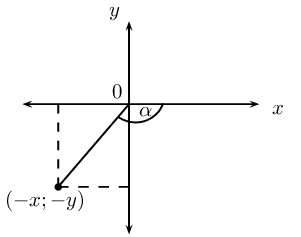$$\cos (-\alpha) = -\cos \alpha$$

If $$t = \sin \text{43}\text{°}$$, express the following in terms of $$t$$:

$$\sin \text{317}\text{°}$$

\begin{align*} \sin \text{317}\text{°}&=\sin(\text{360}\text{°}-\text{43}\text{°}) \\ &=-\sin\text{43}\text{°} \\ &=-t \end{align*}

$$\cos^2 \text{403}\text{°}$$

\begin{align*} \cos^2 \text{403}\text{°}&=\cos^2(\text{360}\text{°}+\text{43}\text{°}) \\ &=\cos^2 \text{43}\text{°} \\ &=1-\sin^2 \text{43}\text{°} \\ &= 1-t^2 \end{align*}

$$\tan (-\text{43}\text{°})$$

\begin{align*} \tan (-\text{43}\text{°}) &= -\tan\text{43}\text{°} \\ &=-\frac{\sin\text{43}\text{°}}{\cos\text{43}\text{°}} \\ &=\pm\frac{t}{\sqrt{1-t^2}} \end{align*}

## Reduction formulae for function values of $$\text{90}\text{°}±\theta$$

In any right-angled triangle, the two acute angles are complements of each other, $$\hat{A} + \hat{C} = \text{90}\text{°}$$Complete the following:

In $$\triangle ABC$$

\begin{align*} \sin \hat{C} &= \dfrac{c}{b} = \cos \ldots \\ \cos \hat{C} &= \dfrac{a}{b} = \sin \ldots \end{align*}

Complementary angles are positive acute angles that add up to $$\text{90}\text{°}$$. For example $$\text{20}\text{°}$$ and $$\text{70}\text{°}$$ are complementary angles.

In the figure $$P(\sqrt{3};1)$$ and $$P'$$ lie on a circle with radius $$\text{2}$$. $$OP$$ makes an angle of $$\theta =\text{30}\text{°}$$ with the $$x$$-axis.1. Function values of $$\text{90}\text{°}-\theta$$

1. If points $$P$$ and $$P'$$ are symmetrical about the line $$y = x$$, determine the coordinates of $$P'$$.

2. Use the coordinates for $$P'$$ to determine $$\sin (\text{90}\text{°} - \theta)$$ and $$\cos(\text{90}\text{°} - \theta)$$.

3. From your results determine a relationship between the function values of $$\left(\text{90}\text{°}-\theta \right)$$ and $$\theta$$.

2. Function values of $$\text{90}\text{°}+\theta$$

In the figure $$P(\sqrt{3};1)$$ and $$P'$$ lie on the circle with radius $$\text{2}$$. $$OP$$ makes an angle $$\theta =\text{30}\text{°}$$ with the $$x$$-axis.1. If point $$P$$ is rotated through $$\text{90}\text{°}$$ to get point $$P'$$, determine the coordinates of $$P'$$.

2. Use the coordinates for $$P'$$ to determine $$\sin (\text{90}\text{°} + \theta)$$ and $$\cos(\text{90}\text{°} + \theta)$$.

3. From your results determine a relationship between the function values of $$\left(\text{90}\text{°}+\theta \right)$$ and $$\theta$$.

3. Complete the following reduction formulae:

1. $$\sin (\text{90}\text{°} - \theta) = \ldots \ldots$$
2. $$\cos (\text{90}\text{°} - \theta) = \ldots \ldots$$
3. $$\sin (\text{90}\text{°} + \theta) = \ldots \ldots$$
4. $$\cos (\text{90}\text{°} + \theta) = \ldots \ldots$$

Sine and cosine are known as co-functions. Two functions are called co-functions if $$f\left(A\right)=g\left(B\right)$$ whenever $$A+B=\text{90}\text{°}$$ (that is, $$A$$ and $$B$$ are complementary angles).

The function value of an angle is equal to the co-function of its complement.

Thus for sine and cosine we have

\begin{align*} \sin(\text{90}\text{°} - θ) &= \cos θ \\ \cos(\text{90}\text{°} - θ) &= \sin θ \end{align*}

The sine and cosine graphs illustrate this clearly: the two graphs are identical except that they have a $$\text{90}\text{°}$$ phase difference.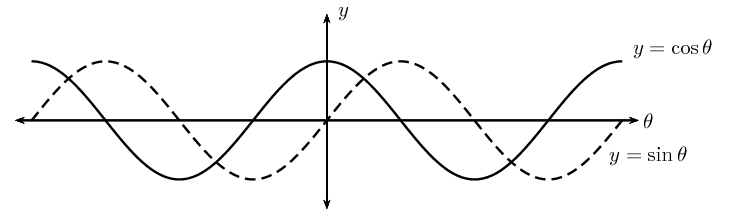## Worked example 10: Using the co-function rule

Write each of the following in terms of $$\sin \text{40}\text{°}$$:

1. $$\cos \text{50}\text{°}$$
2. $$\sin \text{320}\text{°}$$
3. $$\cos \text{230}\text{°}$$
4. $$\cos \text{130}\text{°}$$
1. $$\cos \text{50}\text{°} = \sin ( \text{90}\text{°} - \text{50}\text{°}) = \sin \text{40}\text{°}$$
2. $$\sin \text{320}\text{°} = \sin (\text{360}\text{°} - \text{40}\text{°}) = -\sin \text{40}\text{°}$$
3. $$\cos \text{230}\text{°} = \cos (\text{180}\text{°} + \text{50}\text{°}) = -\cos \text{50}\text{°} = -\cos (\text{90}\text{°} - \text{40}\text{°}) = -\sin \text{40}\text{°}$$
4. $$\cos \text{130}\text{°} = \cos ( \text{90}\text{°} + \text{40}\text{°}) = -\sin \text{40}\text{°}$$

Function values of $$\theta -\text{90}\text{°}$$

We can write $$\sin (\theta - \text{90}\text{°})$$ as

\begin{align*} \sin (\theta - \text{90}\text{°}) &= \sin \left[-(\text{90}\text{°} - \theta)\right] \\ &= -\sin (\text{90}\text{°} - \theta) \\ &= -\cos\theta \end{align*}

similarly, we can show that $$\cos\left(\theta-\text{90}\text{°}\right)=\sin\theta$$

Therefore, $$\sin\left(\theta-\text{90}\text{°}\right)=-\cos\theta$$ and $$\cos\left(\theta-\text{90}\text{°}\right)=\sin\theta$$.

## Worked example 11: Co-functions

Express the following in terms of $$t$$ if $$t = \sin \theta$$:

$\frac{\cos (\theta - \text{90}\text{°})\cos(\text{720}\text{°} + \theta) \tan(\theta - \text{360}\text{°})}{\sin^2(\theta + \text{360}\text{°}) \cos(\theta + \text{90}\text{°})}$

### Simplify the expression using reduction formulae and co-functions

Use the CAST diagram to check in which quadrants the trigonometric ratios are positive and negative.

\begin{align*} &\frac{\cos (\theta - \text{90}\text{°})\cos(\text{720}\text{°} + \theta) \tan(\theta - \text{360}\text{°})}{\sin^2(\theta + \text{360}\text{°}) \cos(\theta + \text{90}\text{°})} \\ =& \frac{\cos [- (\text{90}\text{°} - \theta)] \cos [2(\text{360}\text{°}) + \theta] \tan [-(\text{360}\text{°} - \theta)]}{\sin^2(\text{360}\text{°} + \theta) \cos(\text{90}\text{°} + \theta)} \\ =& \frac{\sin \theta \cos \theta \tan \theta }{\sin^2\theta (-\sin\theta)} \\ =& -\frac{\cos \theta \left( \frac{\sin \theta}{\cos \theta} \right)}{\sin^2\theta} \\ =& - \frac{1}{\sin \theta} \\ =& - \frac{1}{t} \end{align*}

## Co-functions

Textbook Exercise 6.5

Simplify the following:

$$\dfrac{\cos(\text{90}\text{°} + \theta) \sin (\theta + \text{90}\text{°})}{\sin (-\theta)}$$

\begin{align*} \dfrac{\cos(\text{90}\text{°} + \theta) \sin (\theta + \text{90}\text{°})}{\sin (-\theta)} &= \dfrac{-\sin\theta \cos\theta}{-\sin\theta} \\ &= \cos\theta \end{align*}

$$\dfrac{2\sin(\text{90}\text{°} - x) + \sin (\text{90}\text{°} + x)}{\sin (\text{90}\text{°} - x) + \cos(\text{180}\text{°} + x)}$$

\begin{align*} \dfrac{2\sin(\text{90}\text{°} - x) + \sin (\text{90}\text{°} + x)}{\sin (\text{90}\text{°} - x) + \cos(\text{180}\text{°} + x)} &=\dfrac{2\cos x+\cos x}{\cos x+\cos x} \\ &=\dfrac{3\cos x}{2\cos x} \\ &= \frac{3}{2} \end{align*}

Given $$\cos \text{36}\text{°} = p$$, express the following in terms on $$p$$:

$$\sin \text{54}\text{°}$$

\begin{align*} \sin \text{54}\text{°} &=\sin (\text{90}\text{°}-\text{36}\text{°}) \\ &=\cos \text{36}\text{°} \\ &=p \end{align*}

$$\sin \text{36}\text{°}$$

\begin{align*} \sin \text{36}\text{°} &= \sqrt{1 - \cos^2 \text{36}\text{°}} \\ &= \sqrt{1-p^2} \end{align*}

$$\tan \text{126}\text{°}$$

\begin{align*} \tan \text{126}\text{°} &=\frac{\sin(\text{90}\text{°}+\text{36}\text{°})}{\cos(\text{90}\text{°}+\text{36}\text{°})} \\ &=\frac{\cos\text{36}\text{°}}{-\sin\text{36}\text{°}} \\ &=-\frac{p}{\sqrt{1-p^2}} \end{align*}

$$\cos \text{324}\text{°}$$

\begin{align*} \cos \text{324}\text{°} &=\cos(\text{360}\text{°}-\text{36}\text{°}) \\ &=\cos\text{36}\text{°}\\ &=p \end{align*}

Reduction formulae and co-functions:

1. The reduction formulae hold for any angle $$\theta$$. For convenience, we assume $$\theta$$ is an acute angle ($$\text{0}\text{°}<\theta <\text{90}\text{°}$$).

2. When determining function values of ($$\text{180}\text{°}±\theta$$), ($$\text{360}\text{°}±\theta$$) and ($$-\theta$$) the function does not change.

3. When determining function values of ($$\text{90}\text{°}±\theta$$) and ($$\theta ±\text{90}\text{°}$$) the function changes to its co-function.

 second quadrant $$(\text{180}\text{°}-\theta)$$ or $$(\text{90}\text{°}+\theta)$$ first quadrant $$(\theta)$$ or $$(\text{90}\text{°}-\theta)$$ $$\sin(\text{180}\text{°}-\theta)=+\sin\theta$$ all trig functions are positive $$\cos(\text{180}\text{°}-\theta )=-\cos\theta$$ $$\sin(\text{360}\text{°}+\theta )=\sin\theta$$ $$\tan(\text{180}\text{°}-\theta )=-\tan\theta$$ $$\cos(\text{360}\text{°}+\theta )=\cos\theta$$ $$\sin(\text{90}\text{°}+\theta )=+\cos\theta$$ $$\tan(\text{360}\text{°}+\theta )=\tan\theta$$ $$\cos(\text{90}\text{°}+\theta )=-\sin\theta$$ $$\sin(\text{90}\text{°}-\theta )=\cos\theta$$ $$\cos(\text{90}\text{°}-\theta )=\sin\theta$$ third quadrant $$(\text{180}\text{°}+\theta)$$ fourth quadrant $$(\text{360}\text{°}-\theta)$$ $$\sin(\text{180}\text{°}+\theta )=-\sin\theta$$ $$\sin(\text{360}\text{°}-\theta )=-\sin\theta$$ $$\cos(\text{180}\text{°}+\theta )=-\cos\theta$$ $$\cos(\text{360}\text{°}-\theta )=+\cos\theta$$ $$\tan(\text{180}\text{°}+\theta )=+\tan\theta$$ $$\tan(\text{360}\text{°}-\theta )=-\tan\theta$$

## Reduction formulae

Textbook Exercise 6.6

Write $$A$$ and $$B$$ as a single trigonometric ratio:

$$A = \sin(\text{360}\text{°} - \theta) \cos (\text{180}\text{°} - \theta) \tan(\text{360}\text{°} + \theta)$$

\begin{align*} A &= \sin(\text{360}\text{°} - \theta) \cos (\text{180}\text{°} - \theta) \tan(\text{360}\text{°} + \theta) \\ &= ( - \sin \theta )(- \cos \theta )( \tan \theta) \\ &= \sin \theta \cos \theta \frac{\sin \theta}{\cos \theta} \\ &= \sin^2 \theta \end{align*}

$$B = \dfrac{\cos (\text{360}\text{°} + \theta) \cos(-\theta) \sin (-\theta)}{\cos (\text{90}\text{°} + \theta)}$$

\begin{align*} B &= \dfrac{\cos (\text{360}\text{°} + \theta) \cos(-\theta) \sin (-\theta)}{\cos (\text{90}\text{°} + \theta)} \\ &= \dfrac{\cos \theta \cos \theta (- \sin \theta)}{- \sin \theta } \\ &= \dfrac{\cos^2 \theta \sin \theta}{\sin \theta } \\ &= \cos^2 \theta \\ &= \cos^2 \theta \end{align*}

Hence, determine:

$$A + B = \ldots$$

\begin{align*} A + B &= \cos^2 \theta + \sin^2 \theta \\ &= \text{1} \end{align*}

$$\frac{A}{B} = \ldots$$

\begin{align*} \frac{A}{B} &= \frac{\sin^2 \theta }{\cos^2 \theta} \\ &= \tan^2 \theta \end{align*}

Write the following as a function of an acute angle:

$$\sin \text{163}\text{°}$$

\begin{align*} \sin \text{163}\text{°} &= \sin (\text{180}\text{°} - \text{17}\text{°})\\ &= \sin \text{17}\text{°} \end{align*}

$$\cos \text{327}\text{°}$$

\begin{align*} \cos \text{327}\text{°} &= \cos (\text{360}\text{°} - \text{33}\text{°})\\ &= \cos \text{33}\text{°} \end{align*}

$$\tan \text{248}\text{°}$$

\begin{align*} \tan \text{248}\text{°}&= \tan (\text{180}\text{°} + \text{68}\text{°})\\ &= \tan \text{68}\text{°} \end{align*}

$$\cos (-\text{213}\text{°})$$

\begin{align*} \cos (-\text{213}\text{°}) &= \cos \text{213}\text{°} \\ &= \cos (\text{180}\text{°} + \text{33}\text{°})\\ &= - \cos \text{33}\text{°} \end{align*}

Determine the value of the following, without using a calculator:

$$\dfrac{\sin(-\text{30}\text{°})}{\tan(\text{150}\text{°})} + \cos \text{330}\text{°}$$

\begin{align*} \dfrac{\sin(-\text{30}\text{°})}{\tan(\text{150}\text{°})} + \cos \text{330}\text{°} &= \dfrac{- \sin \text{30}\text{°} }{\tan (\text{180}\text{°} - \text{30}\text{°})} + \cos (\text{360}\text{°} - \text{30}\text{°}) \\ &= \dfrac{- \sin \text{30}\text{°} }{- \tan \text{30}\text{°} } + \cos \text{30}\text{°} \\ &= \dfrac{ \sin \text{30}\text{°} }{ \frac{\sin \text{30}\text{°}}{\cos \text{30}\text{°}}} + \cos \text{30}\text{°} \\ &= \cos \text{30}\text{°} + \cos \text{30}\text{°} \\ &= 2 \cos \text{30}\text{°}\\ &= 2 \left( \frac{\sqrt{3}}{2} \right)\\ &= \sqrt{3} \end{align*}

$$\tan \text{300}\text{°} \cos \text{120}\text{°}$$

\begin{align*} \tan \text{300}\text{°} \cos \text{120}\text{°} &= \tan (\text{360}\text{°} - \text{60}\text{°}) \cos (\text{180}\text{°} - \text{60}\text{°}) \\ &= (-\tan \text{60}\text{°})(- \cos \text{60}\text{°}) \\ &= \left( \sqrt{3} \right) \left( \frac{1}{2} \right) \\ &= \frac{\sqrt{3}}{2} \end{align*}

$$(1 - \cos \text{30}\text{°})(1 - \cos \text{210}\text{°})$$

\begin{align*} (1 - \cos \text{30}\text{°})(1 - \cos \text{210}\text{°}) &= (1 - \cos \text{30}\text{°})(1 - \cos (\text{180}\text{°} + \text{30}\text{°})) \\ &= (1 - \cos \text{30}\text{°})(1 + \cos \text{30}\text{°}) \\ &= 1 - \cos^2 \text{30}\text{°} \\ &= \sin^2 \text{30}\text{°} \\ &= \sin^2 \text{30}\text{°} \\ &= \left( \frac{1}{2} \right)^2 \\ &= \frac{1}{4} \end{align*}

$$\cos \text{780}\text{°} - (\sin \text{315}\text{°})(\cos \text{405}\text{°})$$

\begin{align*} &\cos \text{780}\text{°} - (\sin \text{315}\text{°})(\cos \text{405}\text{°}) \\ &= \cos (2(\text{360}\text{°}) + \text{60}\text{°}) - (\sin (\text{360}\text{°} -\text{45}\text{°}))(\cos (\text{360}\text{°} + \text{45}\text{°})) \\ &= \cos\text{60}\text{°} + \sin \text{45}\text{°} \cos\text{45}\text{°} \\ &= \left( \frac{1}{2} \right) + \left( \frac{1}{\sqrt{2}} \right)\left( \frac{1}{\sqrt{2}} \right) \\ &= \left( \frac{1}{2} \right) + \left( \frac{1}{2} \right) \\ &= \text{1} \end{align*}

Prove that the following identity is true and state any restrictions:

$$\dfrac{\sin(\text{180}\text{°} + \alpha) \tan(\text{360}\text{°} + \alpha) \cos \alpha}{\cos(\text{90}\text{°} - \alpha)} = \sin \alpha$$

\begin{align*} \text{LHS } &= \dfrac{\sin(\text{180}\text{°} + \alpha) \tan(\text{360}\text{°} + \alpha) \cos \alpha}{\cos(\text{90}\text{°} - \alpha)} \\ &= \dfrac{(-\sin \alpha) \tan \alpha \cos \alpha}{\sin \alpha } \\ &= -\frac{\sin \alpha}{\cos \alpha} \cos \alpha \\ &= \sin \alpha \\ &= \text{RHS} \end{align*}